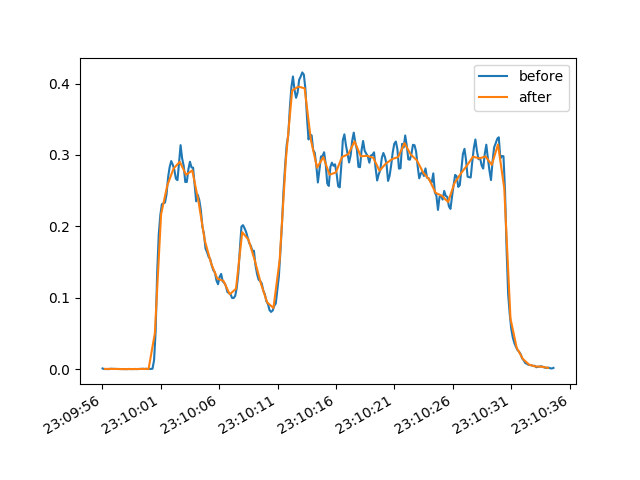## Downsampling and exporting time series data

· ☕ 3 min read · ✍️ Joe

We recently had an email asking about exporting time series data that had been smoothed by averaging. It turns out this is something very easy to do with iolite 4’s built in python interpreter. Before continuing, if you are not familiar with iolite’s python functionality you may want to check out this post first.

### Step by step

Getting access to a channel’s data and time in iolite via python is as easy as:

 1 2  d = data.timeSeries('U238').data() t = data.timeSeries('U238').time() 

This gets us the data/time as NumPy arrays. Now downsampling this data by averaging can be done as follows:

 1 2  import numpy as np ds = np.mean(d.reshape(-1, 4), 1) 

where the 4 means we will average 4 points together. However, this assumes that the length of your data array is divisible by 4. To make things a bit more generic, we could write it as follows:

 1 2 3 4 5  def downsample_for_export(data_array, n): end = n * int(len(data_array)/n) return np.mean(data_array[:end].reshape(-1, n), 1) ds = downsample_for_export(d, 4) 

To demonstrate that this works, we can plot the before and after for a specific selection:Saving the data is also easy using NumPy (delimited, e.g. csv) or pandas (many formats, including Excel):

 1 2 3 4  np.savetxt("/Users/name/Desktop/file.csv", ds, delimiter=",") import pandas as pd pd.DataFrame(data=ds).to_excel("/Users/name/Desktop/file.xlsx", index=False) 

Now suppose we want to be able to specify the amount of time for each data point rather than the number of data points. We could do that by inspecting the time array:

 1 2  tdelta = np.diff(t).mean() n = int(time_per_point/tdelta) 

If we wanted to do it for every channel without needing to specify their names, we could use a for loop as follows:

 1 2 3 4  for channel in data.timeSeriesList(): ds = downsample_for_export(channel.data(), time_per_point) ... 

Lastly, we can also bring in some Qt dependencies to make things a bit more user friendly:

 1 2 3  from iolite.QtGui import QFileDialog, QInputDialog time_per_point = QInputDialog.getDouble(None, "Time per point", "Time per point", 1) filename = QFileDialog.getSaveFileName() 

### Putting it all together

Combining everything above into one neat little script that will ask us what we want for the time per point and where to save the data looks like this:

  1 2 3 4 5 6 7 8 9 10 11 12 13 14 15 16 17 18 19 20 21 22 23 24 25 26 27 28 29 30 31  import numpy as np import pandas as pd from iolite.QtGui import QFileDialog, QInputDialog def downsample_for_export(channel, time_per_point): d = channel.data() t = channel.time() tdelta = np.diff(t).mean() n = int(time_per_point/tdelta) end = n * int(len(d)/n) return (np.mean(t[:end].reshape(-1, n), 1), np.mean(d[:end].reshape(-1, n), 1)) tpoint = QInputDialog.getDouble(None, "Time per point", "Time per point", 1) columns = ["Time"] data_ds = [] for channel in data.timeSeriesList(): t_ds, d_ds = downsample_for_export(channel, tpoint) if not data_ds: data_ds.append(t_ds) columns.append(channel.name) data_ds.append(d_ds) filename = QFileDialog.getSaveFileName() df = pd.DataFrame.from_items(zip(columns, data_ds)) df.to_excel(filename, index=False, sheet_name="Downsampled data") 

Note that now my downsample_for_export function returns a tuple of time and data so that the downsampled time can be used for plotting purposes. Also note that time in iolite is stored as “time since epoch” in seconds. See here for more info, but it is essentially the number of seconds since 1970-01-01 00:00:00.000 UTC.

### How I made the plot

  1 2 3 4 5 6 7 8 9 10 11 12 13 14 15 16 17 18 19 20 21 22  import matplotlib.pyplot as plt import numpy as np import matplotlib.dates as mdate s = data.selectionGroup('Demo').selection(0) U = data.timeSeries('U238').dataForSelection(s) t = data.timeSeries('U238').timeForSelection(s) t = mdate.epoch2num(t) def downsample_for_export(data_array, n): end = n * int(len(data_array)/n) return np.mean(data_array[:end].reshape(-1, n), 1) plt.clf() fig, ax = plt.subplots() plt.plot_date(t, U, "-", label='before') plt.plot_date(downsample_for_export(t, 4), downsample_for_export(U, 4),"-", label='after') date_formatter = mdate.DateFormatter("%H:%M:%S") ax.xaxis.set_major_formatter(date_formatter) fig.autofmt_xdate() plt.legend() plt.savefig('/Users/japetrus/Desktop/demo.png')